## Two events are observed in a frame of reference S to occur at the same space point, the second occurring 1.80 s after the first. In a frame

Question

Two events are observed in a frame of reference S to occur at the same space point, the second occurring 1.80 s after the first. In a frame S′ moving relative to S, the second event is observed to occur 2.05 s after the first. What is the difference between the positions of the two events as measured in S^?

in progress 0
6 months 2021-07-29T02:16:06+00:00 1 Answers 95 views 0

The difference between the positions of the two events as measured in = 3.53 *10^8 m/s

Explanation:

As we know –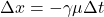Here,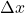is the difference between the positions of the two events as measured in S^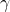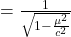And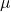= 0.547 c

Substituting the given values in above equation, we get –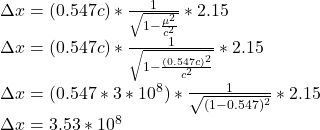meter per second